Next: 3D Motion Model - Up: Representation Previous: Internal Geometry and Projection

### 3D Structure Model

We assume that N feature points are to be tracked over F frames in an image sequence. In the first frame, each feature point'' is initially in terms of an image location (u,v). Subsequent frames are then observed and the image location of the feature is measured with some noisy zero-mean Gaussian error. One may think of the 3D structure of the model as the true (XC,YC,ZC) coordinates of each of the 3D points which then project into (u,v) 2D coordinates. However, this obvious parameterization is not compact and stable. For one thing, it contains 3 unknowns (or 3 degrees of freedom) per point being tracked resulting in the concatenation of 3 x N dimensions to our internal state vector x. Another problem with this representation is that it in no way encodes the rigidity of the object being tracked.

A more compact representation of the 3D location is shown in Equation 14 below. Here, there is only one degree of freedom per point,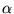. The first term represents the initial image location and the second term represents the perspective ray scaled by the unknown depth: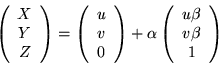(14)

Point-wise structure, therefore, can be represented with one parameter per point (instead of 3).

This representation is consistent with early analyses such as , but inconsistent with representations used in much of the image sequence work, including [10,42,60], which use three parameters per point. It is critical for estimation stability, however, either to use this basic parameterization or to understand and properly handle the additional parameters. Here we describe the computational implications of our parameterization and in Section VII.D we show how it relates to alternate parameterizations.

First consider the total number of unknowns that are to be recovered in a batch solution of these F frames and N points. There are 6(F-1) motion parameters5and 3N structure parameters. Point measurements contribute 2NF constraints and one arbitrary scale constraint must be applied. Hence, the problem can be solved uniquely when 2NF+1 > 6(F-1)+3N. Thus all motion and structure parameters can in principle be recovered from any batch of images for which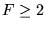and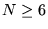.

However, in a recursive solution, not all constraints are applied concurrently. At each step of computation, one frame of measurements constrains all of the structure parameters and one set of motion parameters, i.e. 2N measurements constrain 6+3N degrees of freedom at each frame. This is always an under-determined computation having the undesirable property that the more features that are added, the more under-determined it is. Unless one already has low prior uncertainty on the structure (effectively reducing the dimensionality of unknowns), one should expect unstable and unpredictable estimation behavior from such a formulation. Indeed, in , it was proposed that such filters only be used for tracking'' after a batch procedure is applied for initialization.

On the other hand, in our formulation, constraints (1+2N) outnumber degrees of freedom (6+1+N) for motion, camera, and structure at every frame when N>7. The more measurements available the larger the gap. Our experiments verify that the overdeterminancy results in better stability, allowing for good convergence and tracking in most cases without the requirement of good prior information. In both types of formulation, once structure (and camera, in our case) has converged, each step is effectively overconstrained; the only issue is stability when structure (and camera) is grossly uncertain.

It is clear, then, that excess parameters are undesirable for stability, but how can both 3N- and N-parameter representations describe the same structure? Section VII.D relates the two and demonstrates that when measurement biases are exactly zero (or known) the 3N space really only has N degrees of freedom. Even in the presence of bias, most uncertainty remains along these N DOFs, justifying the structure parameterization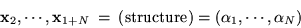We show experimentally that even relatively large biases do not have a strong adverse effect on accuracy using this more concise model.Next: 3D Motion Model - Up: Representation Previous: Internal Geometry and Projection

1999-05-17